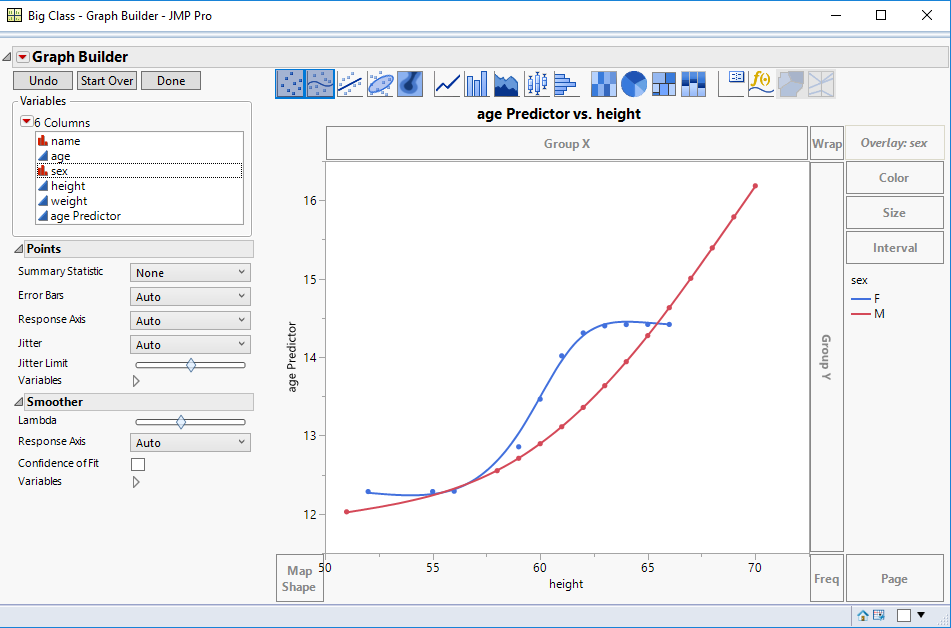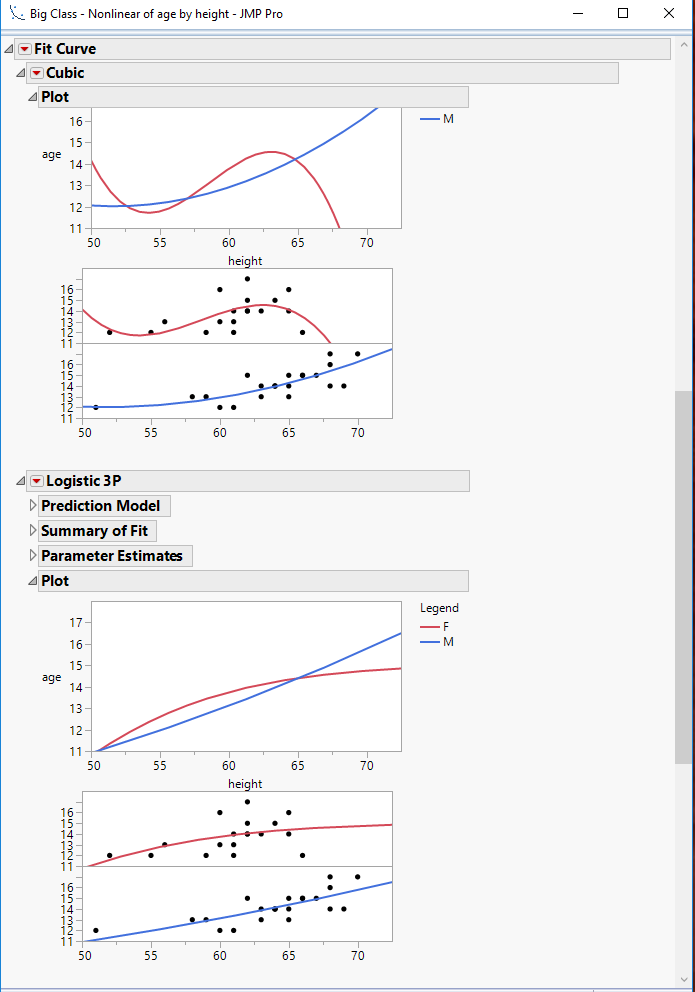Choose Language Hide Translation Bar
Highlighted

## Non-linear modeling, plotting issue

Hi all,

I performed the nonlinear modeling on a data set which is divided in 2 groups by a label.

The modeling works just fine, but is it possible to have the 2 fit curves of the dataset on the same graph?

I've tried with the option Group but it doesn't work...

What can I do?

Thank you!

2 ACCEPTED SOLUTIONS

Accepted Solutions
Highlighted

## Re: Non-linear modeling, plotting issue

You should be able to use Graph Builder, specifying the predictor(formula) column as the Y column, your X column as specified, and then use your grouping column as the overlay column.

If I am still not getting you where you need to go, could you provide some sample data?  I am not a Nonlinear expert, so if I am missing the finer points..........Jim
Highlighted

## Re: Non-linear modeling, plotting issue

Remember to right-click in the Graph Builder plot and select Smoother > Change to > Formula. (Assuming that you save the fitted nonlinear model as a column formula.)

Learn it once, use it forever!
6 REPLIES 6
Highlighted

## Re: Non-linear modeling, plotting issue

Can you provide more specifics,

The version of JMP

The Nlin method you are using

Below is a run of the Nonlinear platform, of age by height, grouped by sex, and it is showing the fit for both sexes on the same graph, in 2 different formats.Jim
Highlighted

## Re: Non-linear modeling, plotting issue

I'm using the Nonlinear fitting on JMP14.

To fit the data I created a new column where to store as formula the model, because it's not in the default fitting type. I'm using this to perform the fitting and obtained the parameters.

Highlighted

## Re: Non-linear modeling, plotting issue

You should be able to use Graph Builder, specifying the predictor(formula) column as the Y column, your X column as specified, and then use your grouping column as the overlay column.

If I am still not getting you where you need to go, could you provide some sample data?  I am not a Nonlinear expert, so if I am missing the finer points..........Jim
Highlighted

## Re: Non-linear modeling, plotting issue

Remember to right-click in the Graph Builder plot and select Smoother > Change to > Formula. (Assuming that you save the fitted nonlinear model as a column formula.)

Learn it once, use it forever!
Highlighted

## Re: Non-linear modeling, plotting issue

Thank you! very much this combined with the advice from @markbailey below helped me to solve this!
Highlighted

## Re: Non-linear modeling, plotting issue

For using a grouping variable, parameters for each level has to be defined in the formula editor (use checkbox "Expand Into Categories..."). However, the plot option will be greyed out, even if the formula is properly defined for a grouping variable.

So within the nonlinear platform report it appears not to be possible to plot more than one curve (JMP 14.2). Jim's suggestion to use Graphbuilder is a good alternative and could probably be automated with JSL.

Article Labels

There are no labels assigned to this post.